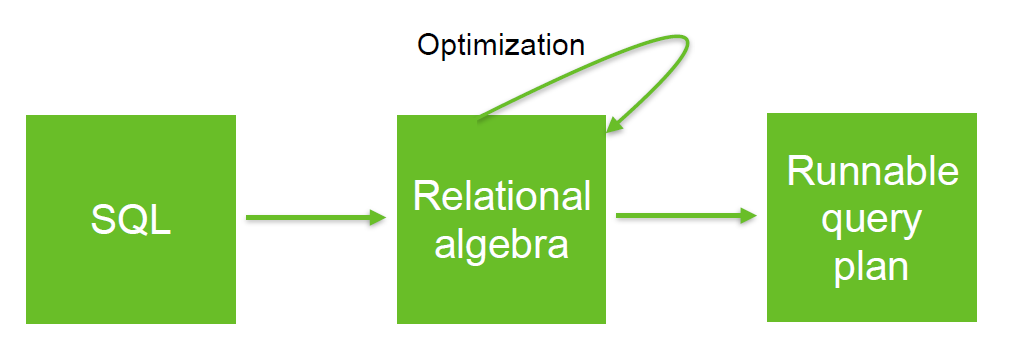# Relational Operator - Rename

Rename or (renaming) is a relational operator that rename:

• a relation
• or an attribute of the relation

## Syntax

$$\LARGE \rho_{a/b} R$$ rename the attribute a from R to b

in SQL, the rename operator is called as

select a as b from R


## Example

Rename the 4 attributes {A, B, A, C} resulting from the cross product between R1 and R2 to {A_1, B, A_2, C} $$\LARGE \rho_{A_1,B,A_2,C} \left( \pi_{A,B}R_1 \times \pi_{A,C}R_2 \right)$$ where:

Discover MoreRelational Algebra - Expression and Operators

Relational algebra is based upon the fact that you can pass tabular data through a set of data operators (select, filter, join, sort, union, etc.) in a algebraic structure. It means that: the output...SQL - As clause

The as sql clause renames a relation or the attribute of a relation. This is a runtime operation and does not rename a table or a column. To rename a table or a column, the alter statement should be...